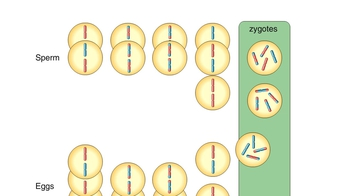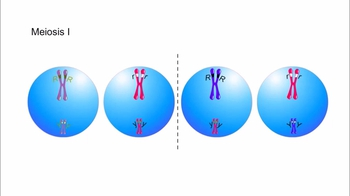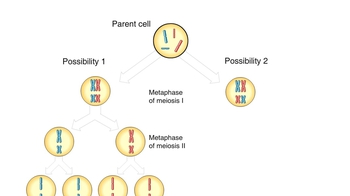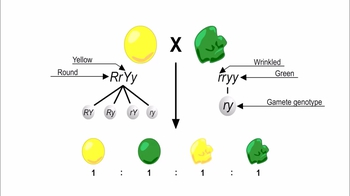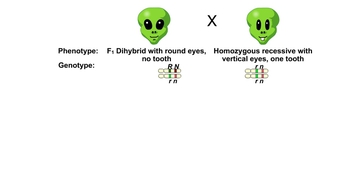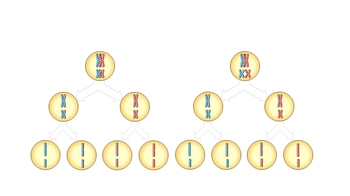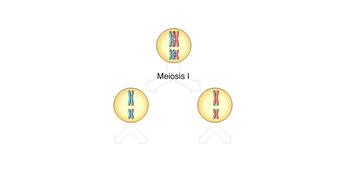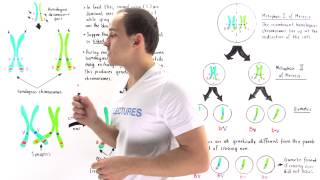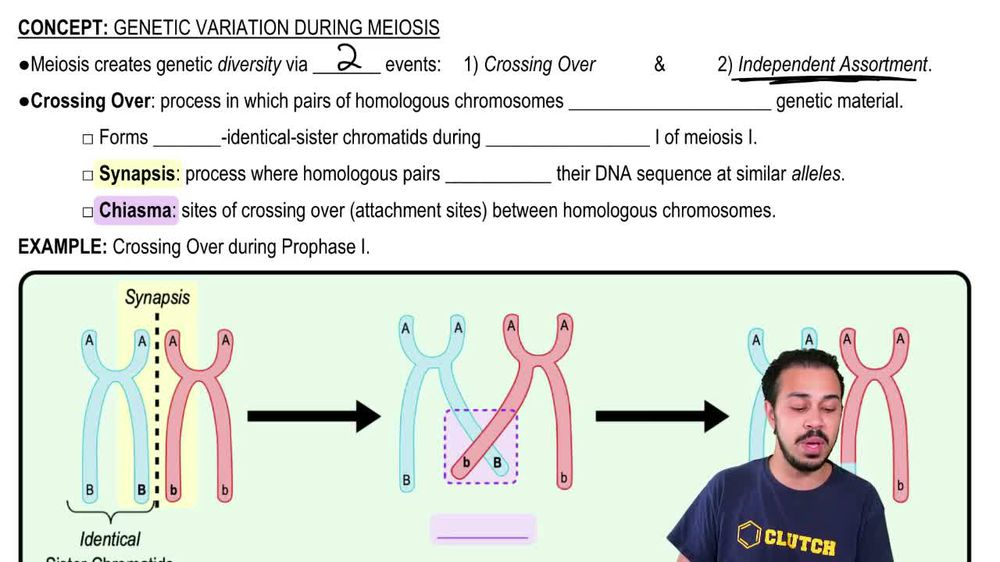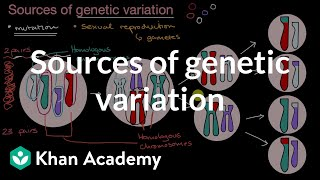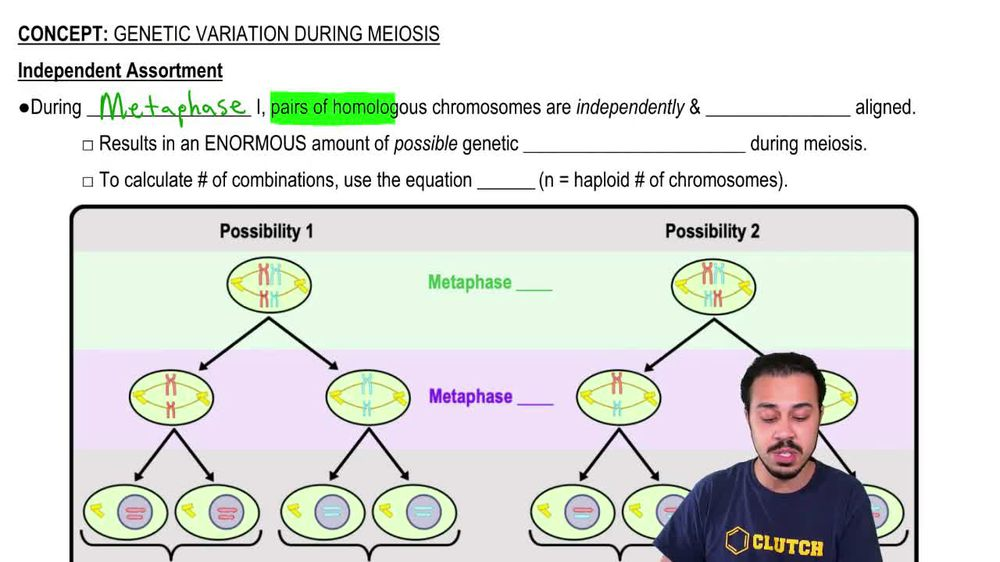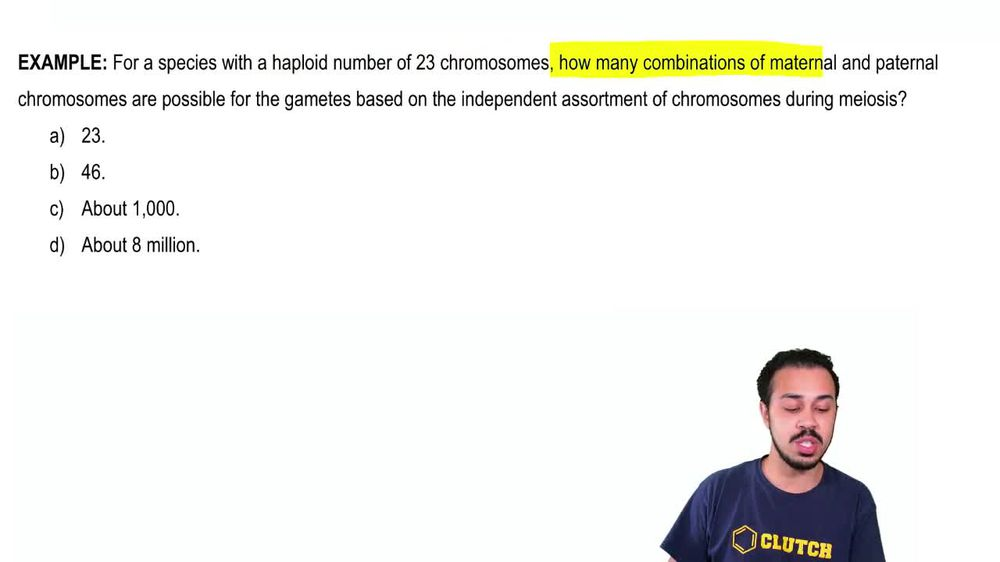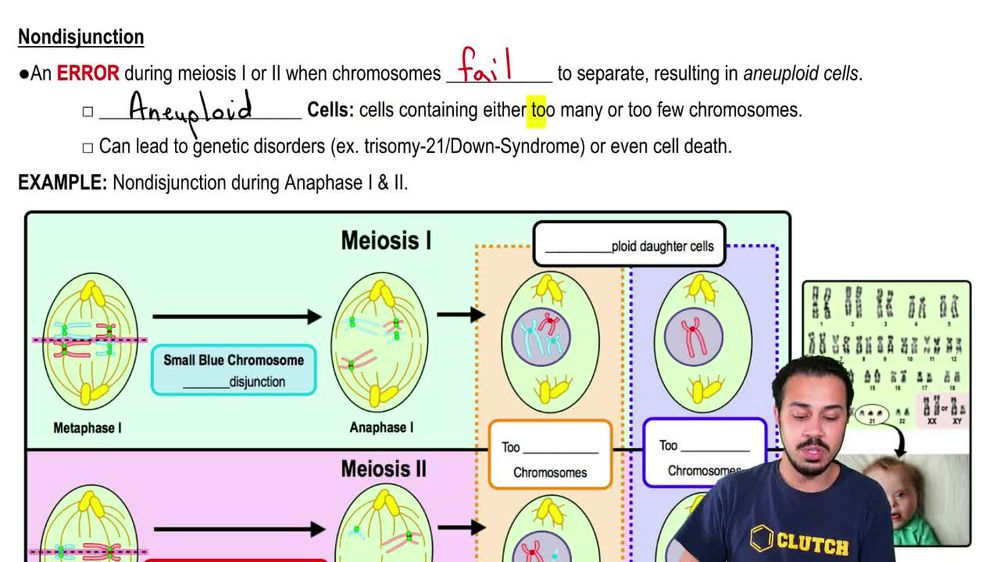Start typing, then use the up and down arrows to select an option from the list.
1. 12. Meiosis2. Genetic Variation During Meiosis# Genetic Variation During Meiosis Example 1

by Jason Amores Sumpter
166 views
1
1
All right. So here we have an example. Problem that says for a species with AHAP Lloyd number of chromosomes, which would be humans. Humans have a hap Lloyd number of 23 chromosomes. How many combinations of maternal and paternal chromosomes are possible for the game? It's based on the independent assortment of chromosomes during my ASUs, and we've got these four potential answer options down below. So really, what this problem is trying to ask us is how maney possible genetic combinations are there If the Hap Lloyd number of chromosomes is 23. And so what we need to recall from our last lesson video is that there is an equation to calculate the number of possible genetic combinations due to independent assortment. And so that equation is right here. The number of possible genetic combinations due to independent assortment is equal to two raised to the power of n where n is equal to the Hap Lloyd number of chromosomes. And so, for this equation, all we need to do is take two and raise it to the power of end again, the Hap Lloyd number of chromosomes. And we're told that the hap Lloyd number of chromosomes is 23 so to raise to the 23 when you type this into your calculator to raise to the power of 23 you'll get an answer of eight million, aN:aN:000NaN 388, possible genetic combinations. And this is a lot of possible genetic combinations when it comes to just independent assortment on its own. And this is every single time my ASUs occurs. And so the likelihood that these four gam it sells that result are going to have the same genetic combination is really unlikely if there are eight million, 388,608 possible genetic combinations. And so, of course, when we look at the answer options option, D says about eight million. And of course, that is going to be the closest one to the correct answer here. So Option D here is going to be the correct answer to this example, and that concludes this example. So I'll see you all in our next video